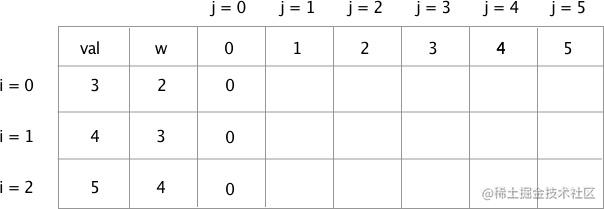# 详解动态规划01背包问题--JavaScript实现## 分析

• 物品无法拆成分数形式，如果能拆分，那就属于贪婪算法问题，在后面的文章我们也会介绍贪婪算法。
• 不一定恰好装满背包。
• 装满时总价值不一定最大。
• 每样物品各一件
• 常规情况下，在表格中，价格和重量一般都是从上到下递增的。我们填表分析的时候，其实是事先默认了这种递增的关系。

### 1. 总容量为0的情况### 2. 第0行，i = 0 的空格分析

`i=0 j=1 ` : 背包总容量为1，但是物品0 的重量为 2，无法装下去，所以这一格应该填 0

`i=0 j=2 ` : 背包总容量为2，刚好可以装下物品0 ，由于物品0 的价值为3，因此这一格填 3

`i=0 j=3 ` : 背包总容量为3，由于根据上面说明的物品组合原则，第0行，仅能放物品0，不需要考虑物品1 和 物品2，所以这一格填 3

`i=0 j=4 ` : 同理，填 3

`i=0 j=5 ` : 同理，填 3

## 3. 第1行，i = 1 的空格分析

`i=1 j=2 ` : 背包总容量为2，只能装下物品0，所以填 3

`i=1 j=3 ` : 背包总容量为3，这时候可以装下一个物品1，或者一个物品0，仅仅从人工填表的方式，很容易理解要选择物品1，但是我们该如何以一个确切的逻辑来表达，让计算机明白呢？基于上面说说明的价值和重量在表格中从上到下递增原则，可以确认物品1的价值是大于物品0的，所以默认情况下优先考虑物品1，当选择了物品1之后，把背包剩余的容量和物品1之前的物品重量对比（也就是和物品0的重量对比，如果剩余重量能装下前面的物品，那么就继续装）。所以这里选择物品1，填 4

`i=1 j=4 ` : 选择了物品1之后，物品1 的重量为3，背包容量为4， 减去物品1的重量后， 剩余容量为1，无法装下物品0，所以这里填 4

`i=1 j=5 ` 选择了物品1之后，剩余的容量为2，刚好可以装下物品0，所以一格背包装了物品1，和物品 0，总价值为7，把 7 填入表格。

## 3. 第2行，i = 2 的空格分析

`i=2 j=1 ` : 填 0

`i=2 j=2 ` : 填写这一行时，3种物品都有机会被装入背包。总容量为2时，只能装物品0，所以填 3

`i=2 j=3 ` : 物品2的重量为4，大于容量j，所以这里可以参考 T[i-1][j]的值，也就是 `i=1 j=3`那一格的值，填 4

`i=2 j=4 ` : 可以装下物品2，价值为5。也可以装下物品1。这一空格需要谨慎一点。我们将使用更严谨的方式来分析。在`i=1 j=5 `中出现了物品组合一起装入背包的情况，这一空将延续这种分析方式。我们选择了物品2，剩余的容量表达式应为 j-w[i]4 - 4 = 0，剩余的容量用于上一行的搜索，由于上一行我们是填写完的，所以可以很轻易地得到这个值。表达式可以写成 `val[i] + T[i-1][j-w[i]]` ，可以根据这个表达式得出一个值。但是这并不是最终结果，还需要和上一行同一列数值对比，即 `T[i-1][j]`，对比，取最大值。最后这里填 5

`i=2 j=5 ` : 根据上面计算原理，这里如果选择了物品2，那么最大价值只能5，参照上一行，同一列，价值为7，取最大值。所以放弃物品2，选择将物品0和物品1装入背包，填写7。

## 伪代码表达

``````if(j < w[i]){ //容量小于重量，hold不住
T[i][j] = T[i-1][j]; //所以值等于上一行，同一列。如果i=0,没有上一行，则T[i][j] 取0
}else{
T[i][j] = max(val[i] + T[i-1][j-w[i]] , T[i-1][j]);  //参照上面 i=2 j=4 和 i=2 j=5 时的填表分析
}

## JavaScript 实现

``````function knapSack(w,val,capacity,n){
var T = []

for(let i = 0;i < n;i++){
T[i] = [];
for(let j=0;j <= capacity;j++){
if(j === 0){ //容量为0
T[i][j] = 0;
continue;
}
if(j < w[i]){ //容量小于物品重量，本行hold不住
if(i === 0){
T[i][j] = 0; // i = 0时，不存在i-1，所以T[i][j]取0

}else{
T[i][j] = T[i-1][j]; //容量小于物品重量，参照上一行

}
continue;
}
if(i === 0){
T[i][j] = val[i]; //第0行，不存在 i-1, 最多只能放这一行的那一个物品
}else{
T[i][j] = Math.max(val[i] + T[i-1][j-w[i]],T[i-1][j]);

}
}

}

findValue(w,val,capacity,n,T);

return T;
}
![](https://p1-jj.byteimg.com/tos-cn-i-t2oaga2asx/gold-user-assets/2018/5/19/16377bff6e2d5c63~tplv-t2oaga2asx-image.image)

//找到需要的物品
function findValue(w,val,capacity,n,T){

var i = n-1, j = capacity;
while ( i > 0 && j > 0 ){

if(T[i][j] != T[i-1][j]){
console.log('选择物品'+i+',重量：'+ w[i] +',价值：' + values[i]);
j = j- w[i];
i--;
}else{
i--;  //如果相等，那么就到 i-1 行
}
}
if(i == 0 ){
if(T[i][j] != 0){ //那么第一行的物品也可以取
console.log('选择物品'+i+',重量：'+ w[i] +',价值：' + values[i]);

}
}
}

// w = [2,3,4].  val = [3,4,5] , n = 3 , capacity = 5
//function knapSack([2,3,4],[3,4,5],5,3);
//
var values = [3,4,5],
weights = [2,3,4],
capacity = 5,
n = values.length;

console.log(knapSack(weights,values,capacity,n));